# Three-day trip

The cyclist on three-day trip travel 30% of the total route on the first day, 3/5 of the rest on the second day and 35 km on the third day. How many kilometers did travel cyclists each day and how many?

a =  37.5
b =  52.5
c =  35
s =  125

### Step-by-step explanation:

a + b + c = s
a = 30/100 s
b = 3/5 • (s-a)
c = 35

a + b + c = s
a = 30/100•s
b = 3/5 • (s-a)
c = 35

a+b+c-s = 0
100a-30s = 0
3a+5b-3s = 0
c = 35

a = 75/2 = 37.5
b = 105/2 = 52.5
s = 125

Our linear equations calculator calculates it.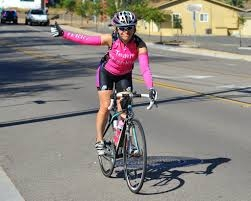Did you find an error or inaccuracy? Feel free to write us. Thank you!Tips to related online calculators
Need help to calculate sum, simplify or multiply fractions? Try our fraction calculator.
Do you have a linear equation or system of equations and looking for its solution? Or do you have a quadratic equation?

## Related math problems and questions:

• The hikerThe hiker will travel 40% of the route on the first day 1and/3 of the rest of the second day. Last day 30 km. What was the length of the 3-day trip? How many kilometers did he walk each day?
• Cyclist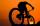Cyclist rode two-fifths of the way the first day. The second day drove 5 km less while traveled three-eighths of route. How many kilometers does he have to finish target?
• Cyclo tripEighth-graders took a full-day trip on a cycling course. They took 1/7 of the route to the first break and added another 3/7 of the route to lunch. They had 18 km left to the finish. How many kilometers did the trip route measure?
• Scouts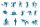Three scouts went on a three day trip. The second day went 4 km more than the first day. The third day went two times less than the first day. They went along 54 km. How many kilometers went every day together?
• Two cyclists 2At the same time, two cyclists left the towns A and B at constant speeds. The first one going from town A to town B, and the second one from town B to town A. At one point of the trip they met. After they met, the first cyclist arrived at town B in 36min,
• Hiking tripRosie went on a hiking trip. The first day she walked 18kilometers. Each day since she walked 90 percent of what she walked the day before. What is the total distance Rosie has traveled by the end of the 10th day? Round your final answer to the nearest ki
• Average speed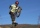The average speed of a good cyclist is 30 km/h. The average speed of the less able is 20 km/h. They both set off on the same route at the same time. Good cyclist drove it 2 hours earlier. How long was the route?
• Cyclists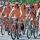Cyclist who rides at an average speed 16 km/h travels trip distance 10 min before the cyclist who rides at an average speed 11 km/h. What is the length of this cyclist trip(distance in km)?
• Motion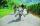Cyclist started at 9:00 from point S to point T. After 10 minutes, followed him at the same speed the second cyclist. Walker, which went from T to S, started at 9:35. After 71 minutes he met the first cyclist and after next 8 minutes the second cyclist. D
• 2 cyclists and carOne cyclist rides at a constant speed over a bridge. It is 100 meters long. When he is 40 meters behind him, he meets an oncoming cyclist who is riding at the same speed. The car travels along the bridge in the same direction as the first cyclist at a spe
• Cycling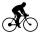Cyclist goes on a trip. First, drove 4 hours average speed 44 kilometers per hour, then slowed down and another 4 hours driving a speed 36 kilometers per hour. How many total kilometers cycling?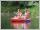Students of canoeists trip traveled in three days 102 km. The second day traveled 15% more than the first day and at the third day 9 km over the second day. How many kilometers are traveled each day?The distance between cities A and B is 132 km. At 9.00 am, the cyclist started the bike at an average speed of 24 km/h, and at 10.00 am started from the B cyclist at an average speed of 30 km/h. How long and far from A will they both meet?The tourist traveled 78km in 3 hours. Part of the journey went on foot at 6km/h, the rest of the trip by bus at 30km/h. How long did he walk?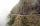Pedestrian goes for a walk first at the plane at 4 km/h, then uphill 3 km/h. Then it is in the middle of the route, turns back, and goes downhill at speed 6 km/h. The total walk was 6 hours. How many kilometers went pedestrian?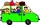On day one of the trip they drove 1/4 of the total distance. On day two they drove 1/2 of the total distance. What fraction of the total distance is left to travel?From two different locations distant 232 km started against car and bus. The car started at 5:20 with average speed 64 km/h. Bus started at 7:30 with average speed 80 km/h. When they meet? How many kilometers went the bus?